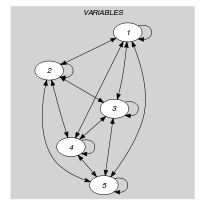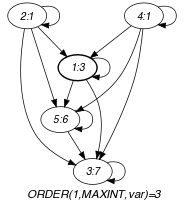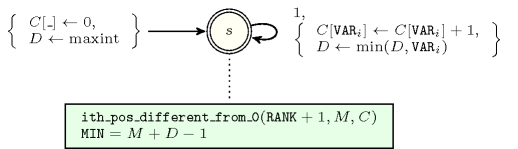## 5.256. min_n

Origin
Constraint

$\mathrm{𝚖𝚒𝚗}_𝚗\left(\mathrm{𝙼𝙸𝙽},\mathrm{𝚁𝙰𝙽𝙺},\mathrm{𝚅𝙰𝚁𝙸𝙰𝙱𝙻𝙴𝚂}\right)$

Arguments
 $\mathrm{𝙼𝙸𝙽}$ $\mathrm{𝚍𝚟𝚊𝚛}$ $\mathrm{𝚁𝙰𝙽𝙺}$ $\mathrm{𝚒𝚗𝚝}$ $\mathrm{𝚅𝙰𝚁𝙸𝙰𝙱𝙻𝙴𝚂}$ $\mathrm{𝚌𝚘𝚕𝚕𝚎𝚌𝚝𝚒𝚘𝚗}\left(\mathrm{𝚟𝚊𝚛}-\mathrm{𝚍𝚟𝚊𝚛}\right)$
Restrictions
 $|\mathrm{𝚅𝙰𝚁𝙸𝙰𝙱𝙻𝙴𝚂}|>0$ $\mathrm{𝚁𝙰𝙽𝙺}\ge 0$ $\mathrm{𝚁𝙰𝙽𝙺}<|\mathrm{𝚅𝙰𝚁𝙸𝙰𝙱𝙻𝙴𝚂}|$ $\mathrm{𝚛𝚎𝚚𝚞𝚒𝚛𝚎𝚍}$$\left(\mathrm{𝚅𝙰𝚁𝙸𝙰𝙱𝙻𝙴𝚂},\mathrm{𝚟𝚊𝚛}\right)$
Purpose

$\mathrm{𝙼𝙸𝙽}$ is the minimum value of rank $\mathrm{𝚁𝙰𝙽𝙺}$ (i.e., the ${\mathrm{𝚁𝙰𝙽𝙺}}^{th}$ smallest distinct value, identical values are merged) of the collection of domain variables $\mathrm{𝚅𝙰𝚁𝙸𝙰𝙱𝙻𝙴𝚂}$. The minimum value has rank 0.

Example
$\left(3,1,〈3,1,7,1,6〉\right)$

The $\mathrm{𝚖𝚒𝚗}_𝚗$ constraint holds since its first argument $\mathrm{𝙼𝙸𝙽}=3$ is fixed to the second (i.e., $\mathrm{𝚁𝙰𝙽𝙺}+1$) smallest distinct value of the collection $〈3,1,7,1,6〉$. Note that identical values are only counted once: this is why the minimum of order 1 is 3 instead of 1.

Typical
 $\mathrm{𝚁𝙰𝙽𝙺}>0$ $\mathrm{𝚁𝙰𝙽𝙺}<3$ $|\mathrm{𝚅𝙰𝚁𝙸𝙰𝙱𝙻𝙴𝚂}|>1$ $\mathrm{𝚛𝚊𝚗𝚐𝚎}$$\left(\mathrm{𝚅𝙰𝚁𝙸𝙰𝙱𝙻𝙴𝚂}.\mathrm{𝚟𝚊𝚛}\right)>1$
Symmetries
• Items of $\mathrm{𝚅𝙰𝚁𝙸𝙰𝙱𝙻𝙴𝚂}$ are permutable.

• One and the same constant can be added to $\mathrm{𝙼𝙸𝙽}$ as well as to the $\mathrm{𝚟𝚊𝚛}$ attribute of all items of $\mathrm{𝚅𝙰𝚁𝙸𝙰𝙱𝙻𝙴𝚂}$.

Arg. properties

Functional dependency: $\mathrm{𝙼𝙸𝙽}$ determined by $\mathrm{𝚁𝙰𝙽𝙺}$ and $\mathrm{𝚅𝙰𝚁𝙸𝙰𝙱𝙻𝙴𝚂}$.

Algorithm
Reformulation

The constraint $\mathrm{𝚊𝚖𝚘𝚗𝚐}_\mathrm{𝚟𝚊𝚛}$$\left(1,〈\mathrm{𝙼𝙸𝙽}〉,\mathrm{𝚅𝙰𝚁𝙸𝙰𝙱𝙻𝙴𝚂}\right)$ enforces $\mathrm{𝙼𝙸𝙽}$ to be assigned one of the values of $\mathrm{𝚅𝙰𝚁𝙸𝙰𝙱𝙻𝙴𝚂}$. The constraint $\mathrm{𝚗𝚟𝚊𝚕𝚞𝚎}$$\left(\mathrm{𝙽𝚅𝙰𝙻},\mathrm{𝚅𝙰𝚁𝙸𝙰𝙱𝙻𝙴𝚂}\right)$ provides a hand on the number of distinct values assigned to the variables of $\mathrm{𝚅𝙰𝚁𝙸𝙰𝙱𝙻𝙴𝚂}$. By associating to each variable ${V}_{i}$ $\left(i\in \left[1,|\mathrm{𝚅𝙰𝚁𝙸𝙰𝙱𝙻𝙴𝚂}|\right]\right)$ of the $\mathrm{𝚅𝙰𝚁𝙸𝙰𝙱𝙻𝙴𝚂}$ collection a rank variable ${R}_{i}\in \left[0,|\mathrm{𝚅𝙰𝚁𝙸𝙰𝙱𝙻𝙴𝚂}|-1\right]$ with the reified constraint ${R}_{i}=\mathrm{𝚁𝙰𝙽𝙺}⇔{V}_{i}=\mathrm{𝙼𝙸𝙽}$, the inequality ${R}_{i}<\mathrm{𝙽𝚅𝙰𝙻}$, and by creating for each pair of variables ${V}_{i},{V}_{j}$ $\left(i,j the reified constraints

${V}_{i}<{V}_{j}⇔{R}_{i}<{R}_{j}$,

${V}_{i}={V}_{j}⇔{R}_{i}={R}_{j}$,

${V}_{i}>{V}_{j}⇔{R}_{i}>{R}_{j}$,

one can reformulate the $\mathrm{𝚖𝚒𝚗}_𝚗$ constraint in term of $3·\frac{|\mathrm{𝚅𝙰𝚁𝙸𝙰𝙱𝙻𝙴𝚂}|·\left(|\mathrm{𝚅𝙰𝚁𝙸𝙰𝙱𝙻𝙴𝚂}|-1\right)}{2}+1$ reified constraints.

generalisation: $\mathrm{𝚖𝚒𝚗𝚒𝚖𝚞𝚖}$ (absolute minimum replaced by minimum or order $𝚗$).

Keywords
Cond. implications

$•$ $\mathrm{𝚖𝚒𝚗}_𝚗\left(\mathrm{𝙼𝙸𝙽},\mathrm{𝚁𝙰𝙽𝙺},\mathrm{𝚅𝙰𝚁𝙸𝙰𝙱𝙻𝙴𝚂}\right)$

implies $\mathrm{𝚊𝚝𝚕𝚎𝚊𝚜𝚝}$$\left(𝙽,\mathrm{𝚅𝙰𝚁𝙸𝙰𝙱𝙻𝙴𝚂},\mathrm{𝙼𝙸𝙽}\right)$

when  $𝙽=1$.

$•$ $\mathrm{𝚖𝚒𝚗}_𝚗\left(\mathrm{𝙼𝙸𝙽},\mathrm{𝚁𝙰𝙽𝙺},\mathrm{𝚅𝙰𝚁𝙸𝙰𝙱𝙻𝙴𝚂}\right)$

with  $\mathrm{𝚁𝙰𝙽𝙺}=1$

and   $\mathrm{𝚖𝚒𝚗𝚟𝚊𝚕}$$\left(\mathrm{𝚅𝙰𝚁𝙸𝙰𝙱𝙻𝙴𝚂}.\mathrm{𝚟𝚊𝚛}\right)=1$

implies $\mathrm{𝚖𝚒𝚗𝚒𝚖𝚞𝚖}_\mathrm{𝚐𝚛𝚎𝚊𝚝𝚎𝚛}_\mathrm{𝚝𝚑𝚊𝚗}$$\left(\mathrm{𝚅𝙰𝚁}\mathtt{1},\mathrm{𝚅𝙰𝚁}\mathtt{2},\mathrm{𝚅𝙰𝚁𝙸𝙰𝙱𝙻𝙴𝚂}\right)$.

Arc input(s)

$\mathrm{𝚅𝙰𝚁𝙸𝙰𝙱𝙻𝙴𝚂}$

Arc generator
$\mathrm{𝐶𝐿𝐼𝑄𝑈𝐸}$$↦\mathrm{𝚌𝚘𝚕𝚕𝚎𝚌𝚝𝚒𝚘𝚗}\left(\mathrm{𝚟𝚊𝚛𝚒𝚊𝚋𝚕𝚎𝚜}\mathtt{1},\mathrm{𝚟𝚊𝚛𝚒𝚊𝚋𝚕𝚎𝚜}\mathtt{2}\right)$

Arc arity
Arc constraint(s)
$\bigvee \left(\begin{array}{c}\mathrm{𝚟𝚊𝚛𝚒𝚊𝚋𝚕𝚎𝚜}\mathtt{1}.\mathrm{𝚔𝚎𝚢}=\mathrm{𝚟𝚊𝚛𝚒𝚊𝚋𝚕𝚎𝚜}\mathtt{2}.\mathrm{𝚔𝚎𝚢},\hfill \\ \mathrm{𝚟𝚊𝚛𝚒𝚊𝚋𝚕𝚎𝚜}\mathtt{1}.\mathrm{𝚟𝚊𝚛}<\mathrm{𝚟𝚊𝚛𝚒𝚊𝚋𝚕𝚎𝚜}\mathtt{2}.\mathrm{𝚟𝚊𝚛}\hfill \end{array}\right)$
Graph property(ies)
$\mathrm{𝐎𝐑𝐃𝐄𝐑}$$\left(\mathrm{𝚁𝙰𝙽𝙺},\mathrm{𝙼𝙰𝚇𝙸𝙽𝚃},\mathrm{𝚟𝚊𝚛}\right)=\mathrm{𝙼𝙸𝙽}$

Graph model

Parts (A) and (B) of Figure 5.256.1 respectively show the initial and final graph associated with the Example slot. Since we use the $\mathrm{𝐎𝐑𝐃𝐄𝐑}$ graph property, the vertex of rank 1 (without considering the loops) of the final graph is shown in grey.

##### Figure 5.256.1. Initial and final graph of the $\mathrm{𝚖𝚒𝚗}_𝚗$ constraint(a) (b)
Automaton

Figure 5.256.2 depicts the automaton associated with the $\mathrm{𝚖𝚒𝚗}_𝚗$ constraint. Figure 5.256.2 depicts the automaton associated with the $\mathrm{𝚖𝚒𝚗}_𝚗$ constraint. To each item of the collection $\mathrm{𝚅𝙰𝚁𝙸𝙰𝙱𝙻𝙴𝚂}$ corresponds a signature variable ${S}_{i}$ that is equal to 1.

##### Figure 5.256.2. Automaton of the $\mathrm{𝚖𝚒𝚗}_𝚗$ constraint